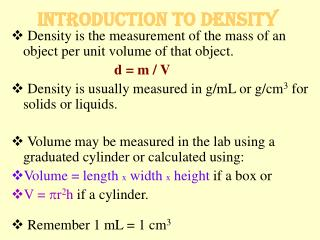DownloadDownload PresentationIntroduction to Density

# Introduction to Density

Download Presentation## Introduction to Density

- - - - - - - - - - - - - - - - - - - - - - - - - - - E N D - - - - - - - - - - - - - - - - - - - - - - - - - - -
##### Presentation Transcript

1. Introduction to Density • Density is the measurement of the mass of an object per unit volume of that object. d = m / V • Density is usually measured in g/mL or g/cm3 for solids or liquids. • Volume may be measured in the lab using a graduated cylinder or calculated using: • Volume = length x width x height if a box or • V = pr2h if a cylinder. • Remember 1 mL = 1 cm3

2. How to measure the density of a solid in the laboratory. • Obtain a clean graduated cylinder. • Fill the graduated cylinder with enough water to cover the object. Record the volume • Carefully place the object into the water filled graduated cylinder. • Record the new water level. • The volume of the object is the Vfinal – Vinitial.

3. Introduction to Density • Example: An object has a mass of 50.0 g and occupies a volume of 52.0 mL. What is its density? d = m / V = 50.0 g / 52.0 mL = 0.98 g/mL • Example: A cylinderical piece of metal is 2.03 inches high and has a diameter 17.0 mm wide and weighs 31.599 g. Identify the metal. V=pr2h = p(1.70 cm/2)2* (2.03 in [2.54 cm/1in]) =11.7 cm3 D = m/V = 31.599 g / 11.7 mL = 2.70 g/mL The metal is aluminum!

4. Practice Problems on density 15.9 mL 1.58 g/mL ___________ 1. Calculate the volume, in microliters, of a cylinder that is 32.42 mm long with a diameter of 0.0789 cm. •  ___________ 2. Calculate the density of a solution if 45.0 mL of the solution weighs 7.12 x 10-2 kg. •  ___________ 3. The Hope diamond weighs 45.52 carats and has a density of 3.51 g/cm3. What is the volume of this diamond? (1 carat = 200 mg) •  ___________ 4. A plastic material weighing 2.2 lb is shaped into a cylinder 13.5 cm in height and 6.8 cm in diameter. Will this object sink or float in water? •  ___________ 5. A student fills a 50.0 mL graduated cylinder with 25.0 mL of water, then places a spherical object weighing 54.367 g into the graduated cylinder. The new volume is 41.5 mL. Calculate the density of the object. 2.59 cm3 2.0 g/cm3; sink 3.29 g/mL